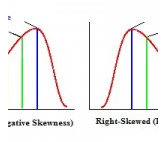Courses

# Kelly’s Measure of Skewness, Business Mathematics & Statistics B Com Notes | EduRev

Created by: Arshit Thakur

## B Com : Kelly’s Measure of Skewness, Business Mathematics & Statistics B Com Notes | EduRev

The document Kelly’s Measure of Skewness, Business Mathematics & Statistics B Com Notes | EduRev is a part of the B Com Course Business Mathematics and Statistics.
All you need of B Com at this link: B Com

## What is Kelly’s Measure of Skewness?

A left-skewed distribution.

Kelly’s Measure of Skewness is one of several ways to measure skewness in a data distribution. Bowley’s skewness is based on the middle 50 percent of the observations in a data set. It leaves 25 percent of the observations in each tail of the distribution. Kelly suggested that leaving out fifty percent of data to calculate skewness was too extreme. He created a measure to find skewness with more data. Kelly’s measure is based on P90 (the 90th percentile) and P10 (the 10th percentile). Only twenty percent of observations (ten percent in each tail) are excluded from the measure.

## Kelly’s Measure Formula.

Kelley’s measure of skewness is given in terms of percentiles and deciles(D). Kelley’s absolute measure of skewness (Sk)is:

Sk=P90 + P10 – 2*P50 = D9 + D1-2*D5.

This formula is not practically used. In fact, Kelly’s measure of skewness is rarely used at all, even in it’s more common form, which is measured as coefficient of skewness:

SP = P90 – 2*P50 + P10 / P90 – P10

What does Kelly’s Measure of Skewness Tell us?

elly’s Measure of Skewness gives you the same information about skewness as the other three types of skewness measures (Bowley skewness, Pearson Mode Skewness and Momental Skewness.).

A measure of skewness = 0 means that the distribution is symmetrical.
A measure of skewness > 0 means a positive skewness.
A measure of skewness < means a negative skewness.

If you prefer an online interactive environment to learn R and statistics, this free R Tutorial by Datacamp is a great way to get started. If you're are somewhat comfortable with R and are interested in going deeper into Statistics, try this Statistics with R track.122 videos|142 docs

,

,

,

,

,

,

,

,

,

,

,

,

,

,

,

,

,

,

,

,

,

,

,

,

;